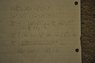# Implicit Differentiation

Sebastian B
I unfortunately keep on getting the wrong answer to this problem.

I am supposed to find: dw/dy(1/(w^2+x^2)+1/(w^2+y^2))

I attached a picture of how I tried to solve it. Help would be much appreciated.

#### Attachments

•_DSC3559.JPG
33.7 KB · Views: 415

Mentor
I unfortunately keep on getting the wrong answer to this problem.

I am supposed to find: dw/dy(1/(w^2+x^2)+1/(w^2+y^2))
No. What you wrote above doesn't mean what you think.
I believe what you are supposed to do is use implicit differentiation to find ##\frac{\partial w}{\partial y}##, although that is not clear from what you wrote on the first line. On the third line you have a mistake. Since ##\frac{1}{w^2 + x^2}## does not involve y, its partial derivative with respect to y is zero.
Sebastian B said:
I attached a picture of how I tried to solve it. Help would be much appreciated.

•Sebastian B
Homework Helper
That 2y should also be part of the multiplication. Double check your application of the chain rule on (w^2+y^2).

Staff Emeritus
Homework Helper
Gold Member
I unfortunately keep on getting the wrong answer to this problem.

I am supposed to find: dw/dy(1/(w^2+x^2)+1/(w^2+y^2))
You mean that you need to find ∂w/∂y given that (1/(w2+x2)+1/(w2+y2)) = 1. Right ?

I attached a picture of how I tried to solve it. Help would be much appreciated.
Hello Sebastian B. Welcome to PF !

In your third equation, you need to have parentheses around ##\displaystyle \ \left( 2w\frac{\partial w}{\partial y}+2y \right) \ ## .

After that the algebra is messed up.

Don't forget; the right side of the equation is zero at that point.

Sebastian B
No. What you wrote above doesn't mean what you think.
I believe what you are supposed to do is use implicit differentiation to find ##\frac{\partial w}{\partial y}##, although that is not clear from what you wrote on the first line. On the third line you have a mistake. Since ##\frac{1}{w^2 + x^2}## does not involve y, its partial derivative with respect to y is zero.

Mark 44: Thank you for your feedback. I realized that I wasn't clear enough with stating my problem, but you figured out what I meant. However,
$\delta$w/$\delta$y (w^2+x^2) does not equal zero, because w could be dependent on y

Sebastian B
Ruber and Sammys thanks a lot for the feedback, it helped a lot! I finally figured it out and got the correct answer thanks to you guys. What a dumb error that was. Thanks again!